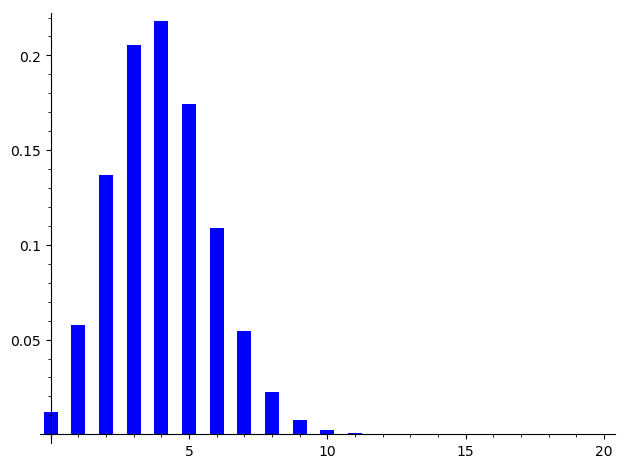# Revision history [back]

The formula can be written in SageMath using binomial, but you may also use binom from the scipy library:

sage: import scipy.stats
sage: binom_dist = scipy.stats.binom(20,.20)
sage: bar_chart([binom_dist.pmf(x) for x in range(20)])As explained in the doc of

sage: scipy.stats.binom?


pmf stands for Probability mass function.

The formula can be written in SageMath using binomial, but you may also use binom from the scipy library:

sage: import scipy.stats
sage: binom_dist = scipy.stats.binom(20,.20)
sage: bar_chart([binom_dist.pmf(x) for x in range(20)])See the Sage quick start for statistics page. As explained in the doc of

sage: scipy.stats.binom?


pmf stands for Probability mass function.

The formula can be written in SageMath using binomial, but you may also use binom from the scipy library:

sage: import scipy.stats
sage: binom_dist = scipy.stats.binom(20,.20)
sage: bar_chart([binom_dist.pmf(x) bar_chart([binom_dist.pmf(k) for x k in range(20)])See the Sage quick start for statistics page. As explained in the doc of

sage: scipy.stats.binom?


pmf stands for Probability mass function.